Open Source Trends Top 20 Best ML Algorithms For Both Newbies and Professionals

# Top 20 Best ML Algorithms For Both Newbies and Professionals

When I started to work with machine learning problems, then I feel panicked which algorithm should I use? Or which one is easy to apply? If you are as like me, then this article might help you to know an overview of machine learning algorithms.

Machine learning is such a powerful AI technique that can perform a task effectively without using any explicit instructions. An ML model can learn from its data and experience. Machine learning applications are automatic, robust and dynamic. Several algorithms are developed to address this dynamic nature of real-life problems. Broadly, there are three types of machine learning algorithms such as supervised learning, unsupervised learning and reinforcement learning.

## 20 Best Machine Learning Algorithms

Selecting the appropriate algorithm is one of the main tasks to develop a machine learning project. Because there are several algorithms are available, and all of them have their benefits and utility. Below we are narrating 20 best ML algorithms for both beginners and professionals. So, let’s take a look.

### 1.  Naive Bayes

A Naïve Bayes classifier is a probabilistic classifier based on Bayes theorem, with the assumption of independence between features. These features differ from application to application. It is one of the most comfortable ML algorithms for beginners to practice.

Naïve Bayes is a conditional probability model. Given a problem instance to be classified, represented by a vector x = (xi . . . xn) representing some n features (independent variables), it assigns to the current instance probabilities for every of K potential outcomes: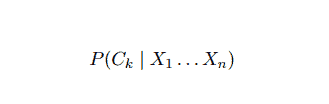The problem with the above formulation is that if the number of features n is significant or if an element can take on a large number of values, then basing such a model on probability tables is infeasible. We, therefore, redevelop the model to make it more tractable. Using Bayes’ theorem, the conditional probability may be written as,Using Bayesian probability terminology, the above equation can be written as:This algorithm is used in text classification, i.e., sentiment analysis, document categorization, spam filtering, and news classification. This algorithm performs well if the input data are categorized into predefined groups. Also, it requires less data than logistic regression. It outperforms in various domain.

### 2. Support Vector Machine

Support Vector Machine (SVM) is one of the most extensively used supervised machine learning algorithms in the field of text classification. This algorithm is also used for regression. It can also be referred to as Support Vector Networks. Cortes & Vapnik developed this method for binary classification. The supervised learning model is the machine learning approach that infers the output from the labeled training data.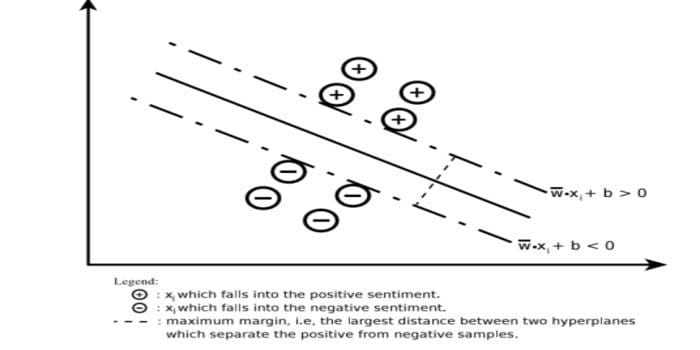A support vector machine constructs a hyperplane or set of hyperplanes in a very high or infinite-dimensional area. It computes the linear separation surface with a maximum margin for a given training set.

Only a subset of the input vectors will influence the choice of the margin (circled in the figure); such vectors are called support vectors. When a linear separation surface does not exist, for example in the presence of noisy data, SVMs algorithms with a slack variable are appropriate. This Classifier attempts to partition the data space with the use of linear or non-linear delineations between the different classes.

SVM has been widely used in pattern classification problems and nonlinear regression. Also, it is one of the best techniques for performing automatic text categorization. The best thing about this algorithm is that it does not make any strong assumptions on data.

To implement Support Vector Machine: data Science Libraries in Python– SciKit Learn, PyML, SVMStruct  Python, LIBSVM and data Science Libraries in R– klar, e1071.

### 3. Linear Regression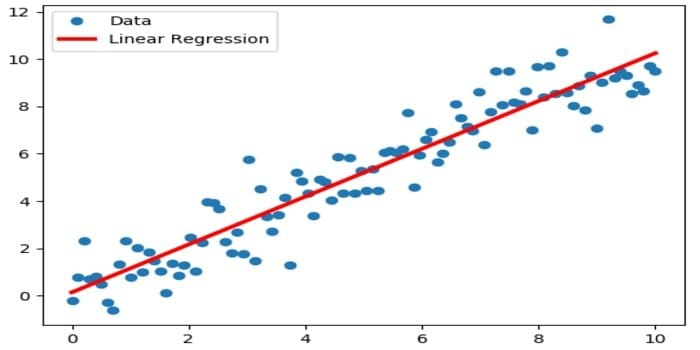Linear regression is a direct approach that is used to modeling the relationship between a dependent variable and one or more independent variables. If there is one independent variable, then it is called simple linear regression. If more than one independent variable is available, then this is called multiple linear regression.

This formula is employed to estimate real values like the price of homes, number of calls, total sales based on continuous variables. Here, the relationship between independent and dependent variables is established by fitting the best line. This best fit line is known as a regression line and represented by a linear equation

Y= a *X + b.

here,

• Y – dependent variable
• a – slope
• X – independent variable
• b – intercept

This algorithm is easy to use. It executes fast. This can be used in business for sales forecasting. It can also be used in risk assessment.

### 4.  Logistic RegressionLogistic regression or logit regression is used to estimate discrete values (Binary values like 0/1, yes/no, true/false) based on a given set of the independent variable. The task of this algorithm is to predict the probability of an incident by fitting data to a logit function. Its output values lie between 0 and 1.

The formula can be used in various areas like machine learning,  scientific discipline, and medical fields. It can be used to predict the danger of occurring a given disease based on the observed characteristics of the patient. Logistic regression can be utilized for the prediction of a customer’s desire to buy a product. This algorithm is used in weather forecasting to predict the probability of having rain.

Logistic regression can be divided into three types –

• Binary Logistic Regression
• Multi-nominal Logistic Regression
• Ordinal Logistic Regression

Logistic regression is less complicated. Also, it is robust. It can handle non-linear effects. However, if the training data is sparse and high dimensional, this algorithm may overfit. It cannot predict continuous outcomes.

### 5. K-Nearest-Neighbor (KNN)

K-nearest-neighbor (kNN) is a well known statistical approach for classification and has been widely studied over the years, and has applied early to categorization tasks. It acts as a non-parametric methodology for classification and regression problems.

The algorithm is quite simple. It determines the category of a test document t based on the voting of a set of k documents that are nearest to t in terms of distance, usually Euclidean distance. The essential decision rule given a testing document t for the kNN classifier is: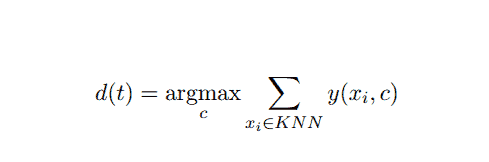Where y (xi,c ) is a binary classification function for training document xi (which returns value 1 if xi is labeled with c, or 0 otherwise), this rule labels with t with the category that is given the most votes in the k-nearest neighborhood.

We can be mapped KNN to our real lives. For example, if you would like to find out a few people, of whom you have got no info, you would possibly prefer to decide regarding his close friends and therefore the circles he moves in and gain access to his/her information. This algorithm is computationally expensive.

### 6. K-means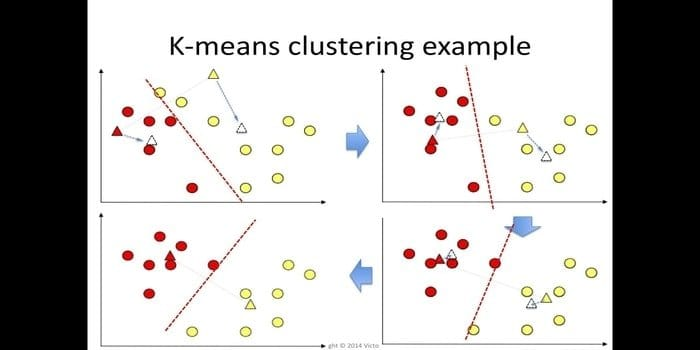k-means clustering is a method of unsupervised learning which is accessible for cluster analysis in data mining. The purpose of this algorithm is to divide n observations into k clusters where every observation belongs to the closest mean of the cluster.  This algorithm is used in market segmentation, computer vision, and astronomy among many other domains.

### 7. Decision Tree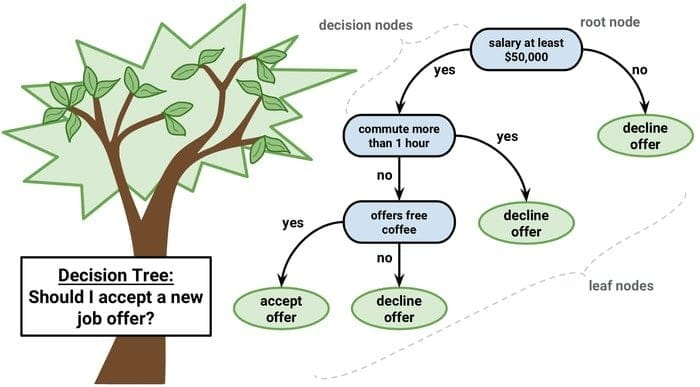A decision tree is a decision support tool that uses a graphical representation, i.e., tree-like graph or model of decisions. It is commonly used in decision analysis and also a popular tool in machine learning. Decision trees are used in operations research and operations management.

It has a flowchart-like structure in which every internal node represents a ‘test’ on an attribute, every branch represents the outcome of the test, and each leaf node represents a class label. The route from the root to leaf is known as classification rules. It consists of three types of nodes:

• Decision nodes: typically represented by squares,
• Chance nodes: typically represented by circles,
• End nodes: typically represented by triangles.

A decision tree is simple to understand and interpret. It uses a white box model. Also, it can combine with other decision techniques.

### 8. Random ForestRandom forest is a popular technique of ensemble learning which operates by constructing a multitude of decision trees at training time and output the category that’s the mode of the categories (classification) or mean prediction (regression) of each tree.

The runtime of this algorithm is fast, and it can able to work with the unbalanced and missing data. However, when we used it for regression, it cannot predict beyond the range in the training data, and it may over-fit data.

### 9. CARTClassification and Regression Tree (CART) is one kind of decision tree. A Decision Tree is working as a recursive partitioning approach and CART divides each of the input nodes into two child nodes. At each level of a decision tree, the algorithm identifies a condition – which variable and level to be used for splitting the input node into two child nodes.

CART algorithm steps are given below:

• Take Input data
• Best Split
• Best Variable
• Split the input data into left and right nodes
• Continue step 2-4
• Decision Tree Pruning

### 10. Apriori Machine Learning Algorithm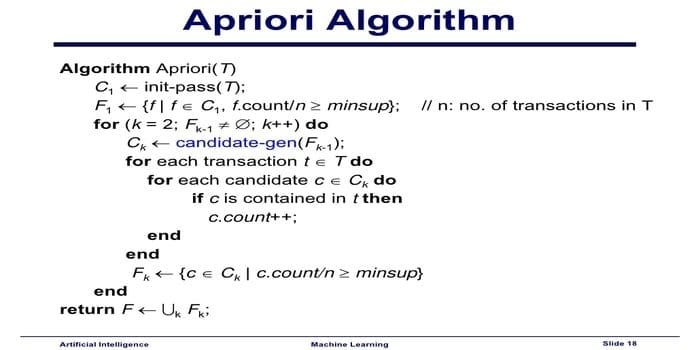The Apriori algorithm is a categorization algorithm. This algorithm is used for sorting large amounts of data. It can also be used to follow up on how relationships develop, and categories are built. This algorithm is an unsupervised learning method that generates association rules from a given data set.

Apriori Machine Learning Algorithm works as:

• If an item set occurs frequently, then all the subsets of the item set also happen often.
• If an item set occurs infrequently, then all the supersets of the item set have also infrequent occurrence.

This algorithm is used in a variety of applications such as to detect adverse drug reactions, for market basket analysis and auto-complete applications. It’s straightforward to implement.

### 11. Principal Component Analysis (PCA)Principal component analysis (PCA) is an unsupervised algorithm. The new features are orthogonal that means they are not correlated. Before performing PCA, you should always normalize your dataset because the transformation is dependent on scale. If you do not, the features that are on the most significant scale will dominate new principal components.

PCA is a versatile technique. This algorithm is effortless and simple to implement. It can be used in image processing.

### 12. CatBoost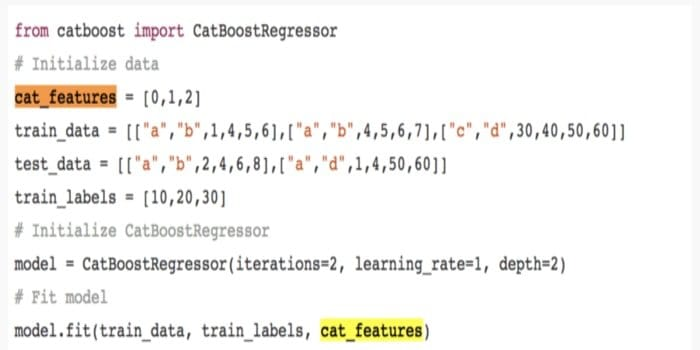CatBoost is an open-sourced machine learning algorithm which comes from Yandex. The name ‘CatBoost’ comes from two words’ Category’ and ‘Boosting.’ It can combine with deep learning frameworks, i.e., Google’s TensorFlow and Apple’s Core ML. CatBoost can work with numerous data types to solve several problems.

### 13. Iterative Dichotomiser 3 (ID3)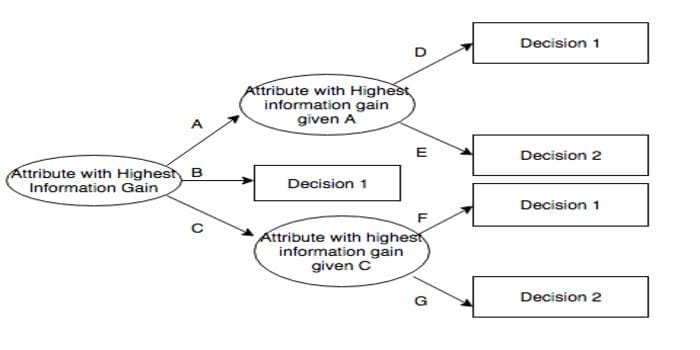Iterative Dichotomiser 3(ID3) is a decision tree learning algorithmic rule presented by Ross Quinlan that is employed to supply a decision tree from a dataset. It is the precursor to the C4.5 algorithmic program and is employed within the machine learning and linguistic communication process domains.

ID3 may overfit to the training data. This algorithmic rule is tougher to use on continuous data. It does not guarantee an optimal solution.

### 14. Hierarchical Clustering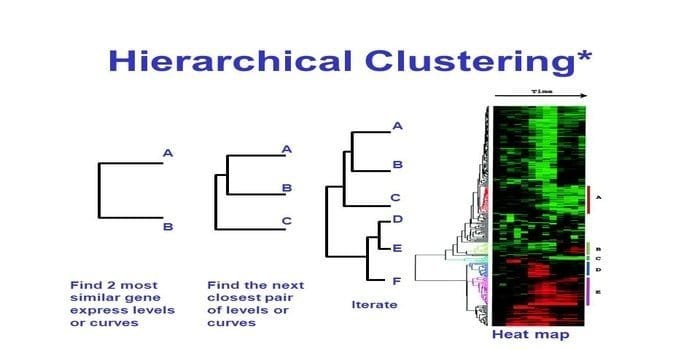Hierarchical clustering is a way of cluster analysis. In hierarchical clustering, a cluster tree (a dendrogram) is developed to illustrate data. In hierarchical clustering, each group (node) links to two or more successor groups. Each node within the cluster tree contains similar data. Nodes group on the graph next to other similar nodes.

#### Algorithm

The algorithms can be divided into two model – bottom up or top down:

Bottom-up (Hierarchical Agglomerative Clustering, HAC)

• At the beginning of the algorithm, take each document as a single cluster.
• In a new cluster, merged two items at a time. How the combines merge involves calculative a difference between every incorporated pair and therefore the alternative samples. There are many options to do this. Some of them are:

a. Complete linkage: Similarity of the furthest pair. One limitation is that outliers might cause the merging of close groups later than is optimal.

b. Single-linkage: The similarity of the closest pair. It may cause premature merging, though those groups are quite different.

c. Group average: similarity between groups.

d. Centroid similarity: each iteration merges the clusters with the foremost similar central point.

• Until all items merge into a single cluster, the pairing process is going on.

Top down (Divisive Clustering)

• Data starts with a combined cluster.
• The cluster divides into two distinct parts, according to some degree of similarity.
• Clusters divide into two again and again until the clusters only contain a single data point.

### 15. Back-PropagationBack-propagation is a supervised learning algorithm. This algorithm comes from the area of  ANN (Artificial Neural Networks). This network is a multilayer feed-forward network. This technique aims to design a given function by modifying the internal weights of input signals to produce the desired output signal. It can be used for classification and regression.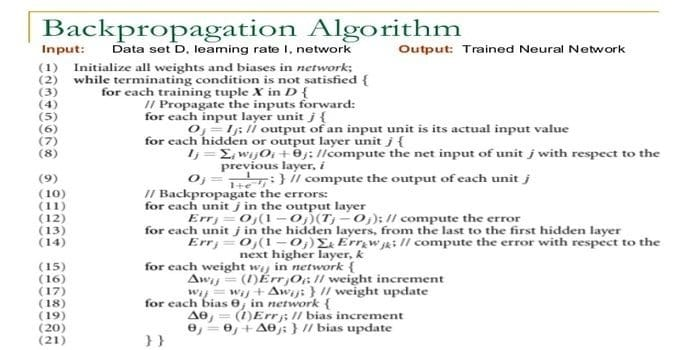Back-propagation algorithm has some advantages, i.e., its easy to implement. The mathematical formula used in the algorithm can be applied to any network. Computation time may be reduced if the weights are small.

Back-propagation algorithm has some drawbacks such as it may be sensitive to noisy data and outliers. It is an entirely matrix-based approach. The actual performance of this algorithm entirely depends on input data. The output may non-numeric.

### 16. AdaBoost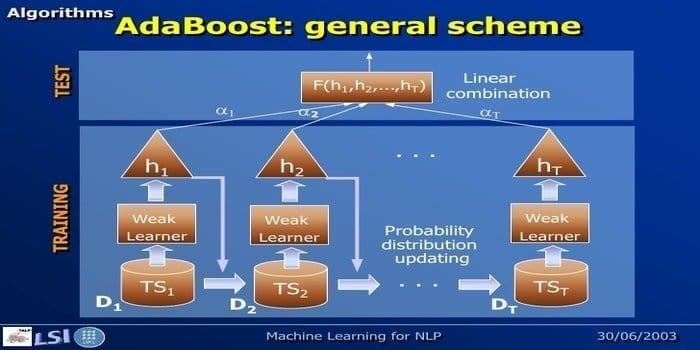AdaBoost means Adaptive Boosting, a machine learning algorithm represented by Yoav Freund and Robert Schapire. It is a meta-algorithm and can be integrated with other learning algorithms to enhance their performance. This algorithm is quick and easy to use. It works well with large data sets.

### 17. Deep LearningDeep learning is a set of techniques inspired by the mechanism of the human brain. The two primary deep learning, i.e., Convolution Neural Networks (CNN) and Recurrent Neural Networks (RNN) are used in text classification. Deep learning algorithms like Word2Vec or GloVe are also employed to get high-ranking vector representations of words and improve the accuracy of classifiers which is trained with traditional machine learning algorithms.

This algorithm needs a lot of training sample instead of traditional machine learning algorithms, i.e., a minimum of millions of labeled examples. On the opposite hand, traditional machine learning techniques reach a precise threshold wherever adding more training sample does not improve their accuracy overall. Deep learning classifiers outperform better result with more data.

### 18. Gradient Boosting Algorithm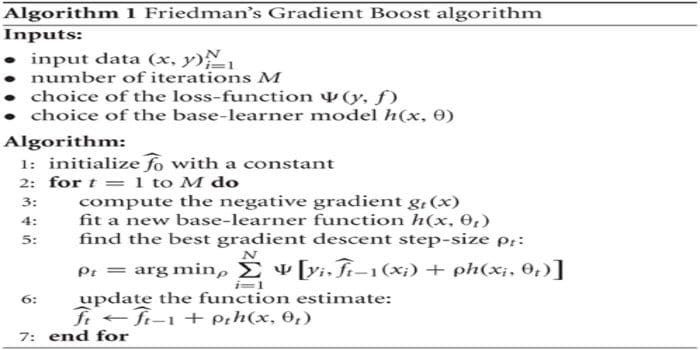Gradient boosting is a machine learning method which is used for classification and regression. It is one of the most powerful ways of developing a predictive model. A gradient boosting algorithm has three elements:

• Loss Function
• Weak Learner
• Additive Model

### 19. Hopfield Network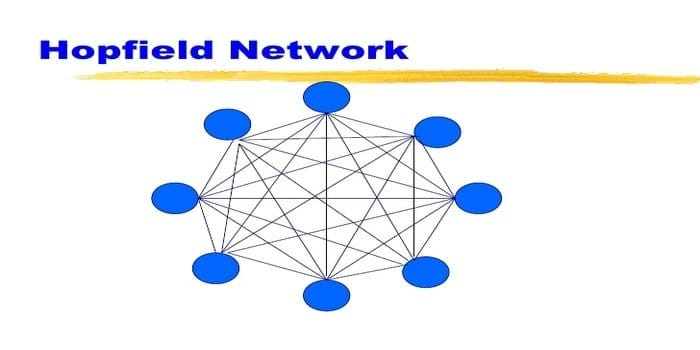A Hopfield network is one kind of recurrent artificial neural network given by John Hopfield in 1982. This network aims to store one or more patterns and to recall the full patterns based on partial input. In a Hopfield network, all the nodes are both inputs and outputs and fully interconnected.

### 20. C4.5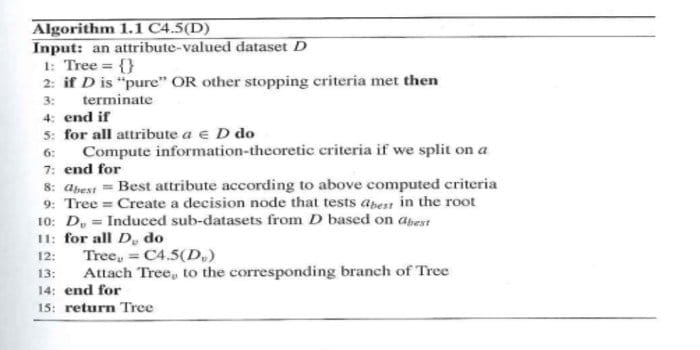C4.5 is a decision tree which is invented by Ross Quinlan. Its an upgrade version of ID3. This algorithmic program encompasses a few base cases:

• All the samples in the list belong to a similar category. It creates a leaf node for the decision tree saying to decide on that category.
• It creates a decision node higher up the tree using the expected value of the class.
• It creates a decision node higher up the tree using the expected value.

## Ending Thoughts

It’s very much essential to use the proper algorithm based on your data and domain to develop an efficient machine learning project. Also, understanding the critical difference between every machine learning algorithm is essential to address ‘when I pick which one.’ As, in a machine learning approach, a machine or device has learned through the learning algorithm. I firmly believe that this article helps you to understand the algorithm. If you have any suggestion or query, please feel free to ask. Keep reading.

### Top 15 Best Disk Cloning Software for Linux in 2019

No matter whether you're a professional sysadmin like me or just an average Linux maniac, you must understand the importance of regularly backing up...

### Top 10 Potential Applications of Machine Learning in Healthcare

With the rapid growth of the population, it seems challenging to record and analyze the massive amount of information about patients. Machine learning provides...

### How To Set Up A VPN With Linux: A Comprehensive Beginner’s Guide

A VPN or a Virtual Private Network basically means creating a secured private network using public infrastructure. This process helps create a protected network...

### Linux or Windows: 25 Things You Must Know While Choosing The Best Platform

Choosing the best platform - Linux or Windows is complicated. Because both the system is versatile and capable of doing many mission-oriented and regular...

### Most Stable Linux Distros: 5 versions of Linux We Recommend

At the very beginning, I would like to mention why the term ‘Stable’ comes in relation to Linux OS or distro. Because there are...

### 15 Best Things To Do After Installing Linux Mint 19 “Tara”

Linux Mint is one of the best Linux distros for newcomers, especially who comes from other Operating Systems like Windows and MacOS. Linux Mint...